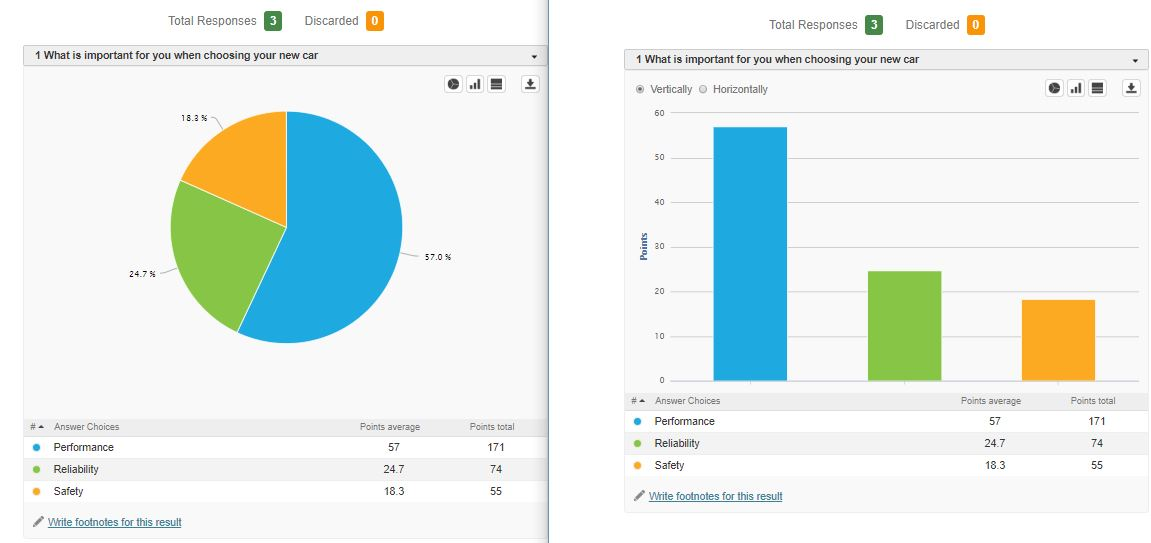Usage

Use if you want the respondent to divide the value (eg points, money, percent) between the options. You will receive the information about how important the options are. The whole value must be divided (all points without any remainder) always.Analyzing results

This question can be evaluated by using the average values (how many points on average people give to given options) and the total sum (the total number of points assigned to the given options).

Graph

Both pie and bar graphs can be used to display the results.Options & Settings

• Set the total value (you want to divide 10, or 100 or 1000 units).
• Set unit values (eg USD, points, percentages).
• Add an image or video to the question.